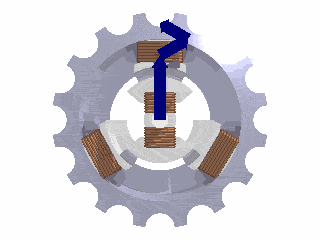Home » Posts tagged 'Electronics and Electrical'

# Tag Archives: Electronics and Electrical

## photodiode sensor

i want to make a sensor using photodiodes. here photodiodes are connecting parallel and to each photodiode i have to put a load resistance. how can we calculate the value of photo diode? on what basis we can design the load resistance value. i am applying a voltage of 2.5 volt . Is there any method for finding the load resistance value of a photo diode ?## Single phase, two phase, three phase and multiphase electric generators – which one is more efficient?

I started referring to the above issue when one students asked, “Why do we use three phase generation of power and not two phase or four phase?”

Three-phase has properties that make it very desirable in electric power systems:

The phase currents tend to cancel out one another, summing to zero in the case of a linear balanced load. This makes it possible to reduce the size of the neutral conductor because it carries little to no current; all the phase conductors carry the same current and so can be the same size, for a balanced load.Vector sum of the magnetic field vectors of the stator coils produces a single rotating vector of resulting rotating magnetic field. (Photo credit: Wikipedia)

Power transfer into a linear balanced load is constant, which helps to reduce generator and motor vibrations.

Three-phase systems can produce a rotating magnetic field with a specified direction and constant magnitude, which simplifies the design of electric motors.

Refer the following links for more …

http://en.wikipedia.org/wiki/Two-phase_electric_power

http://en.wikipedia.org/wiki/Polyphase_system

http://en.wikipedia.org/wiki/Three-phase_electric_power## Current Electricity – Drift Velocity based numerical

Two different wires X and Y of same diameter but different materials are joined together in series     across a battery . If the number density of electrons in X is  twice that in Y . Find ratio of drift velocity of electrons in two wires .

In series combination, the current is the same.

I = nAev

=> n1v1 = n2 v2

=> v1:v2 =n2:n1## TransformersA hypothetical ideal transformer considered as a circuit element, consisting of N P turns in the primary winding, and N S in the secondary. Electrical power supplied to the primary circuit is delivered to the load in secondary circuit by means of mutual induction. The time-varying magnetic flux in the core generates an electromagnetic force over each of the windings. The voltage and current relationships are shown below. (Photo credit: Wikipedia)

Please explain what is transformer and tell what is the reason behind the working of transformer that it reduces potenial when current is high and increases potential when current is low?

Explain widely and deeply with concepts.

Transformer is a device based on mutual inductance and is used to change the voltage of alternating (or varying) current. There are two types of transformers – stepup and stepdown.

A step-up transformer increases the voltage as the step-down transformer decreases the ac voltage.

When a transformer changes the alternating voltage, it is not adding any power, it only transforms the voltage.

As power is the product of voltage and current, as voltage increases, the current decreases. (and vice versa)

## V proportional to I or I proportional to V in Ohm’s Law?

Why we used I proportional to V?
Can we write V proportional to I also?

Both are ok. If we are changing the current and observing the voltage, then it is V∝ I

If we are changing the voltage and observing the current, then

I∝ V

In general, we write

the dependent variable proportional to the independent variable

dependent variable  = the quantity measured by us

independent variable = the quantity fixed by us## Strength of a cable and number of strands

Is the strength of a single strand of a steel cable proportionate to the entire cable? meaning if lets say a cable was made up of 100 strands and could lift a hundred pounds, would each thread be able to lift a pound, or does the cable as a whole become stronger?The strength of the cable increases with increase in the number of strands.## Resistance of Transmission lines

A high voltage transmission line has an aluminum cable of diameter 3.0 cm , 200 km long . What is the resistance of this cable?

Use the relation R = ρ l/A

A= πr2 =πd2/4

= 3.14 x 3 x 10-2x 3 x 10-2/4

ρ= 2.82×10−8 ohm m

l = 200km = 200000 m = 2 x 105m

You can now just substitute the values and get the answer.### Hits so far @ AskPhysics

• 2,171,689 hits

### CBSE Exam Count Down

AISSCE / AISSE 2020March 1, 2019
Board Exams for the core subjects of CBSE may start from 1 March. (Some subjects will have exams in Feb itself)